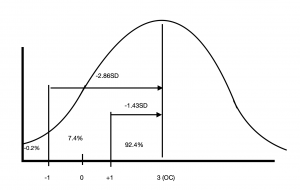# Magnitude-based Bayesian calculator.

ShareThe proposed calculator is intended to assist the practitioner in evaluating physical and physiological tests.

It based on magnitude-based inference method and Bayesian statistics.
For a detailed description of the method, see the article.

In short:
You need to enter following for each test:
1. Observed change (OC) . This is difference between pre-training and post-training tests.
2. Typical error (TE) of the test.
3. Smallest worthwhile change (SWC).
You can learn how to find TE and SWC in the same article.

My suggestion is to make SWC equal to TE.

Based on this input Calculator gives you probability of positive, trivial and negative changes for each test (likelihoods).
Then, if you have a group of tests, the calculator calculates the Bayesian probability. This method allows the result of each new test to update the probabilities.

Multiply by 100 to get the percentage chance (for example, 0.6 probability = 60% chance).

Good luck!

Ctl Test OC TE SWC SD Z(-SWC)  Z(+SWC) Lp Lt Ln

### Instructions.

Enter values for (green colour): Observed Change (OC); Typical Error (TE); SWC and press “Calculate”.

Use the same number of rows as you have tests for analysis. Don’t leave empty rows!

“+” – adding a row below; “-” – deleting a current row.

Output for analysis (red colour):

Likelihoods (Lp;Lt;Ln) – probability of changes in individual tests. Lp- positive likelihood; Lt- trivial likelihood; Ln- negative likelihood.

Bayesian probabilities  – bayesian calculation for group of tests. If there is only one test, then the likelihoods and Bayesian probabilities are the same.

BPP- Bayesian positive probability; BNP- Bayesian negative probability; BTP- Bayesian trivial probability.

Note: Sum of likelihoods and Bayesian probabilities  for all three outcomes (positive+trivial+negative) must be 1. Sometimes Calculator gives 0.99-1.01 due to rounding issue. I think in our case such precision is not a problem.

### Interpretation of results.

A probability of about 0.33 (33%) is the probability “by-chance”. This is not informative. The minimum informative probability is about 0.5 (50%). This is the probability of an outcome that is equal to the other two outcomes. For one test, this is a positive probability when OC=SWC. High probabilities, in my opinion, start at 0.75 (75%). For one test, this is a positive probability when OC=2 SWC and SWC=TE (as I advised).

Share